• ## Dot Product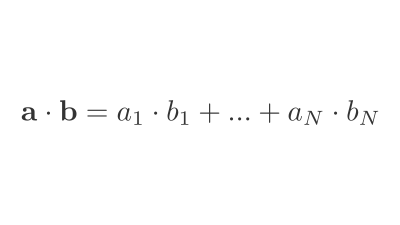An operation between 2 vectors that returns a number.

• ## Binary Cross-Entropy Loss

Binary Cross-Entropy (BCE), also known as log loss, is a loss function used in binary or multi-label machine learning training.

It's nearly identical to Negative …

• ## Presizing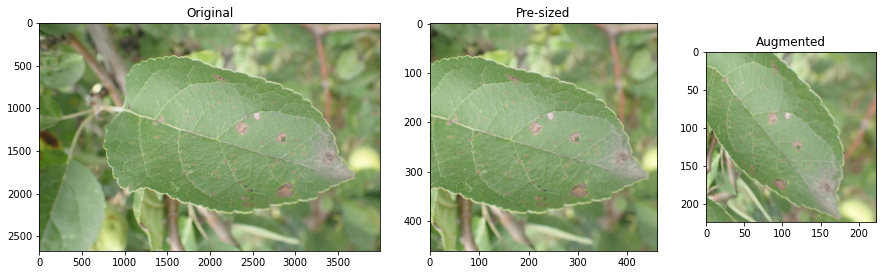Pre-sizing is a technique for preparing images for training a neural network.

In standard image pipelines, images are resized to a size suitable for our …

• ## Categorical Cross-Entropy Loss

Categorical Cross-Entropy Loss Function, also known as Softmax Loss, is a loss function used in multiclass classification model training. It applies the Softmax Activation Function …

• ## Cross-Entropy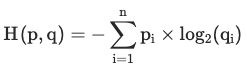Cross-entropy measures the average number of bits required to identify an event if you had a coding scheme optimised for one probability distribution $q …$

• ## Information EntropyEntropy is a measure of uncertainty of a random variable's possible outcomes.

It's highest when there are many equally likely outcomes. As you introduce more …

• ## Negative Log-Likelihood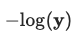Negative log-likelihood is a loss function used in multi-class classification.

Calculated as $-log(\textbf{y})$, where $\mathbf{\text{y …}}$

• ## Softmax Activation Function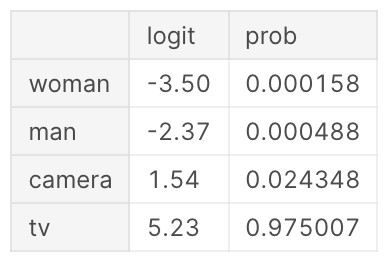The Softmax function converts a vector of numbers into a vector of probabilities that sum to 1. It's applied to a model's outputs (or Logits …

• ## Sigmoid Activation Function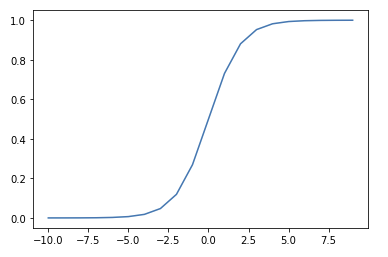The Sigmoid function squeezes numbers into a probability-like range between 0 and 1.1 Used in Binary Classification model architectures to compute loss on discrete …

• ## Domain ShiftWhen production data diverges significantly from the training dataset